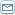# Understanding Pips in Forex Currency Trading

 By: Jason FielderAs a new Forex trader, one of the most important things you will need to learn is how to figure out the value of a pip for any currency pair. A pip is the smallest measure of value in a currency pair in Forex, so it's critical that you understand this concept. When someone is saying "30 pips," they're talking about thirty units of value in a trade. Both profits and losses are measured in pips, though a pip for USD/JPY is not the same value as a pip for USD/CAD. The simplest way to put it is this: one pip is one unit of the smallest measured decimal place. For example, if you are trading USD/JPY at 114.95, then one pip is .01 Yen, since that is the smallest decimal place of measurement used in this pair. The JPY is measured in two decimal spaces, although almost all other currencies are measured in four decimal places. This does vary, which is why you always want to check on each individual currency to figure out what the pips actually are. For example, if the USD/CAD is trading at 1.0621 CAD than a pip for this transaction is .0001 CAD. If you trade AUD/USD while it's at 1.2433, then one pip for this trade is .0001 since that combination has four decimal places, as well. See how that works? Like many parts of Forex trading, it is pretty easy once you get used to it. So if the USD/JPY is quoted to only two decimal places, so Yen .01 is the value of this pip. If this pair goes from 114.95 to 115.00, it gained 5 pips. Likewise, if the USD/CAD goes from 1.0621 to 1.0611, it lost 10 pips. That simple math is all that's needed at that point. So if USD/JPY went from 88.25 to 88.29 that would be a 4 pip increase. On the other hand, if it went from 88.25 to 87.90, that would be a 35 pip loss. The math might seem a little daunting at first, but it really is just ordinary math an easy enough to pick up on. As a side note: many non-Yen currencies are figured out four places, making many pips involving USD in the currency pair .0001, but just remember that a pip is one unit of the furthest listed decimal point and you'll do fine. This also means that for each currency pair the pip can be a different value. You will want to keep track of this.
Most Read Articles On
"Foreign Exchange"SuggestedTop Searches on

 Share this article :
Click to see more related articles